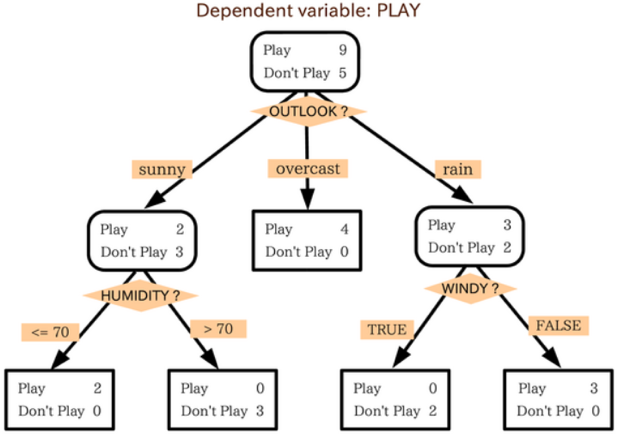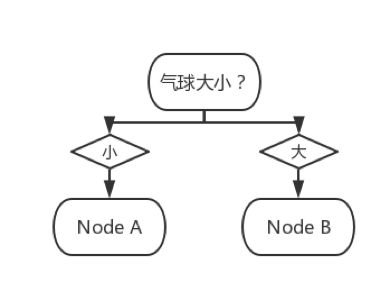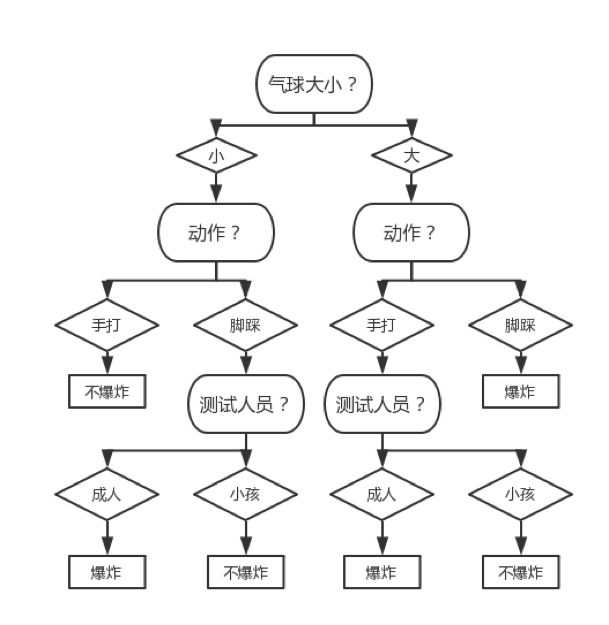# 决策树的相关术语

• 将样本空间划分为若干个互不相交的子空间
• 给每个子空间贴一个类别标签

# 决策树的生成算法

• ID3 算法可说是“最朴素”的决策树算法，它给出了对离散型数据分类的解决方案
• C4.5 算法在其上进一步发展、给出了对混合型数据分类的解决方案
• CART 算法则更进一步、给出了对数据回归的解决方案

• 向根节点输入数据
• 根据信息增益的度量、选择数据的某个特征来把数据划分成（互不相交的）好几份并分别喂给一个新 Node
• 如果分完数据后发现：
• 某份数据的不确定较小、亦即其中某一类别的样本已经占了大多数，此时就不再对这份数据继续进行划分、将对应的 Node 转化为叶节点
• 某份数据的不确定性仍然较大，那么这份数据就要继续分割下去（转第 2 步）

## ID3（Interactive Dichotomizer-3）

ID3 可以译为“交互式二分法”，虽说这个名字里面带了个“二分”，但该方法完全适用于“多分”的情况。它选择互信息作为信息增益的度量、针对离散型数据进行划分。其算法叙述如下：

1. 输入：训练数据集$D = \{\left( x_{1},y_{1} \right),\ldots,(x_{N},y_{N})\}$
2. 过程
1. 将数据集$D$喂给一个 Node
2. $D$中的所有样本同属于类别$c_{k}$，则该 Node 不再继续生成、并将其类别标记为$c_{k}$
3. $x_{i}$已经是 0 维向量、亦即已没有可选特征，则将此时$D$中样本个数最多的类别$c_{k}$作为该 Node 的类别
4. 否则，按照互信息定义的信息增益： 来计算第 j 维特征的信息增益，然后选择使得信息增益最大的特征$x^{\left( j^{*} \right)}$作为划分标准，亦即：
5. $x^{\left( j^{*} \right)}$满足停止条件、则不再继续生成并则将此时$D$中样本个数最多的类别$c_{k}$作为类别标记
6. 否则，依$x^{\left( j^{*} \right)}$的所有可能取值$\{ a_{1},\ldots,a_{m}\}$将数据集$D$划分为${\{ D}_{1},\ldots,D_{m}\}$、使得： 同时，将$x_{1},\ldots,x_{N}$的第$j^{*}$维去掉、使它们成为$n - 1$维的特征向量
7. 对每个$D_{j}$从 2.1 开始调用算法
3. 输出：原始数据对应的 Node（亦即根节点）

• 若选择$x^{\left( j^{*} \right)}$作为特征时信息增益$g(y,x^{\left( j^{*} \right)})$仍然很小（通常会传入一个参数$\epsilon$作为阈值）、则停止
• 事先把数据集分为训练集与测试集（交叉验证的思想），若由训练集得到的$x^{\left( j^{*} \right)}$并不能使得决策树在测试集上的错误率更小、则停止## C4.5

C4.5 使用信息增益比作为信息增益的度量，从而缓解了 ID3 算法会倾向于选择 m 比较大的特征$A$作为划分依据这个问题；也正因如此，C4.5 算法可以处理 ID3 算法比较难处理的混合型数据。我们先来看看它在离散型数据上的算法（仅展示和 ID3 算法中不同的部分）：

### 算法 2.4 步

• 若在当前数据集中有 m 个取值，不妨假设它们为$u_1,...,u_m$；不失一般性、再不妨假设它们满足$u_1<...（若不然，进行一次排序操作即可），那么依次选择$v_1,...,v_p$作为二分标准并决出最好的一个，其中$v_1,...,v_p$构成等差数列、且: p 的选取则视情况而定，一般而言会取 p 反比于“深度”。这意味着当数据越分越细时、对特征的划分会越分越粗，从直观上来说这有益于防止过拟合

• 依次选择$v_{1} = \frac{u_{1} + u_{2}}{2},\ldots,v_{m - 1} = \frac{u_{m - 1} + u_{m}}{2}$作为二分标准、计算它们的信息增益比、从而决出最好的二分标准来划分数据

• $u_1,...,u_m$所对应的类别是$y_1,...,y_m$，那么在$v_1,...,v_{m-1}$中只选取使得： $v_i$作为二分标准、计算它们的信息增益比、从而决出最好的二分标准来划分数据

## CART

CART 的全称是 Classification and Regression Tree（“分类与回归树”）。顾名思义，它既可做分类亦可做回归。囿于篇幅，本书会介绍分类问题的算法和实现、对于回归问题则只叙述算法，感兴趣的观众老爷们可以尝试触类旁通地实现它

CART 算法一般会使用基尼增益作为信息增益的度量（当然也可以使用互信息和信息增益比作为度量，需要视具体场合而定），其一大特色就是它假设了最终生成的决策树为二叉树、亦即它在处理离散型特征时也会通过决出二分标准来划分数据：

### 算法 2.4 步

• $x^{\left( j \right)}$是离散型的，则依次选取$u_{1}^{\left( j \right)},\ldots,u_{S_{j}}^{\left( j \right)}$作为二分标准$a_{p}$，此时：
• $x^{\left( j \right)}$是连续型的，则依次选取$\frac{u_{1}^{\left( j \right)} + u_{2}^{\left( j \right)}}{2},\ldots,\frac{u_{S_{j} - 1}^{\left( j \right)} + u_{S_{j}}^{\left( j \right)}}{2}$作为二分标准$a_{p}$，此时： 按照基尼系数定义的信息增益： 来计算第 j 维特征在这些二分标准下的信息增益，然后选择使得信息增益最大的特征$x^{\left( j^{*} \right)}$和相应的二分标准$u_{p^{*}}^{\left( j^{*} \right)}$作为划分标准，亦即：

• 对于分类问题，模型可表示为：
• 对于回归问题，模型可表示为： 这里$c_{m} = \arg{\min_{c}{L_{m}\left( c \right)}} \triangleq \arg{\min_{c}{\sum_{\left( x_{i},y_{i} \right) \in R_{m}}^{}\left( y_{i} - c \right)^{2}}}$。那么由一阶条件： 可解得$c_{m} = \text{avg}\left( y_{i}|(x_{i},y_{i}) \in R_{m} \right) \triangleq \frac{1}{\left| R_{m} \right|}\sum_{\left( x_{i},y_{i} \right) \in R_{m}}^{}y_{i}$

• 解决分类问题时，我们会在特征和二分标准选好后，通过求解： 来选取划分标准
• 解决回归问题时，我们会在特征和二分标准选好后，通过求解： 来选取划分标准，其中$c_{jp}^{\left( 1 \right)} = \text{avg}(y_{i}|x_{i} < p)$$c_{jp}^{\left( 2 \right)} = \text{avg}(y_{i}|x_{i} \geq p)$，p（切分点）的选取则视情况而定（可以模仿分类问题中二分标准的选取方法）